International
Tables for
Crystallography
Volume B
Reciprocal space
Edited by U. Shmueli

International Tables for Crystallography (2006). Vol. B, ch. 5.1, pp. 545-546   | 1 | 2 |

## Section 5.1.7.1.1. Non-absorbing crystals

A. Authiera*

aLaboratoire de Minéralogie-Cristallographie, Université P. et M. Curie, 4 Place Jussieu, F-75252 Paris CEDEX 05, France
Correspondence e-mail: authier@lmcp.jussieu.fr

#### 5.1.7.1.1. Non-absorbing crystals

| top | pdf |

Rocking curve. The geometrical construction in Fig. 5.1.3.5shows that, in the Bragg case, the normal to the crystal surface drawn from the extremity of the incident wavevector intersects the dispersion surface either at two points of the same branch,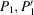, for branch 1,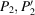for branch 2, or at imaginary points. It was shown in Section 5.1.2.6that the propagation of the wavefields inside the crystal is along the normal to the dispersion surface at the corresponding tie points. Fig. 5.1.3.5shows that this direction is oriented towards the outside of the crystal for tie points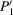and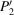. In a very thick crystal, these wavefields cannot exist because there is always a small amount of absorption. One concludes that in the thick-crystal case and in reflection geometry, only one wavefield is excited inside the crystal. It corresponds to branch 1 on the low-angle side of the rocking curve and to branch 2 on the high-angle side. Using the same approximations as in Section 5.1.6.2, the amplitude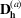of the wave reflected at the crystal surface is obtained by applying the boundary conditions, which are particularly simple in this case: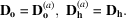The reflecting power is given by an expression similar to (5.1.6.7):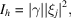where the expression for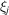is given by (5.1.3.12), and j = 1 or 2 depending on which wavefield propagates towards the inside of the crystal. When the normal to the entrance surface intersects the dispersion surface at imaginary points, i.e. when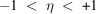,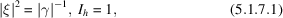and there is total reflection. Outside the total-reflection domain, the reflecting power is given by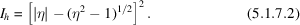The rocking curve has the well known top-hat shape (Fig. 5.1.7.1). Far from the total-reflection domain, the curve can be approximated by the function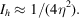Figure 5.1.7.1 | top | pdf |Theoretical rocking curve in the reflection case for a non-absorbing thick crystal in terms of the deviation parameter.

Width of the total-reflection domain. The width of the total-reflection domain is equal to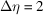and its angular width is therefore equal, using (5.1.3.5), to 2δ, where δ is given by (5.1.3.6). It is proportional to the structure factor, the polarization factor C and the square root of the asymmetry factor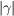. Using an asymmetric reflection, it is therefore possible to decrease the width at wish. This is used in monochromators to produce a pseudo plane wave [see, for instance, Kikuta & Kohra (1970)]. It is possible to deduce the value of the form factor from very accurate measurements of the rocking curve; see, for instance, Kikuta (1971).

Integrated intensity. The integrated intensity is defined by (5.1.6.8):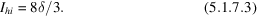Penetration depth. Within the domain of total reflection, there are two wavefields propagating inside the crystal with imaginary wavevectors, one towards the inside of the crystal and the other one in the opposite direction, so that they cancel out and, globally, no energy penetrates the crystal. The absorption coefficient of the waves penetrating the crystal is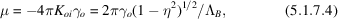where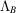is the value taken by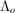[equation (5.1.3.8)] in the Bragg case.

The penetration depth is a minimum at the middle of the reflection domain and at this point it is equal to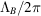. This attenuation effect is called extinction, andis called the extinction length. It is a specific property owing to the existence of wavefields. The resulting propagation direction of energy is parallel to the crystal surface, but with a cross section equal to zero: it is an evanescent wave [see, for instance, Cowan et al. (1986)].

### References

Cowan, P. L., Brennan, S., Jach, T., Bedzyk, M. J. & Materlik, G. (1986). Observations of the diffraction of evanescent X-rays at a crystal surface. Phys. Rev. Lett. 57, 2399–2402.Google Scholar
Kikuta, S. (1971). Determination of structure factors of X-rays using half-widths of the Bragg diffraction curves from perfect single crystals. Phys. Status Solidi B, 45, 333–341.Google Scholar
Kikuta, S. & Kohra, K. (1970). X-ray collimators using successive asymmetric diffractions and their applications to measurements of diffraction curves. I. General considerations on collimators. J. Phys. Soc. Jpn, 29, 1322–1328.Google Scholar